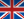Une voie, plusieurs choix
Informatique et Mathématiques appliquées# Fundamentals of Probabilistic data mining - WMM9MO17

A+Augmenter la taille du texteA-Réduire la taille du texteImprimer le documentEnvoyer cette page par mail cet article Facebook Twitter Linked In
• #### Number of hours

• Lectures : 15.0
• Tutorials : -
• Laboratory works : 4.5
• Projects : -
• Internship : -
• Written tests : -
ECTS : 3.0
• Officials : Xavier ALAMEDA-PINEDA

### Goals

This lecture introduces fundamental concepts and associated numerical methods in model-based clustering, classification and models with latent structure. We will also discuss probabilistic graphical models with latent variables in general, the expectation maximisation algorithm and its variational Bayes formulation. Overall we will discuss Gaussian mixture models, hidden Markov models, probabilistic PCA, linear dynamical systems, and more complex models that are combinations of these ones, and that require variational expectation-maximisation algorithms. If time allows, we will discuss the link with variational auto-encoders.

Content

Model-based clustering, classification and models with latent structure are particularly relevant to model random vectors, sequences or graphs, to account for data heterogeneity, and to present general principles in statistical modelling. The following topics are addressed:

Principles of probabilistic data mining and generative models; models with latent variables
Probabilistic graphical models
Mixture models and clustering
PCA and probabilistic PCA
Generative models for series and graphs : hidden Markov models
Linear dynamical systems.
Variational EM algorithm.

Prerequisites

Fundamental principles in probability theory (conditioning) and statistics (maximum likelihood estimator and its usual asymptotic properties).
Constrained optimization, Lagrange multipliers.

Tests

3-hours written exam (E) and one report on practicals and research work (P)
Authorized documents: handwritten notes only.

N1=0.5E1+0.5P
N2=E2

The exam is given in english onlyCalendar

The course exists in the following branches:

• Curriculum - Master 2 in Applied Mathematics - Semester 9 (this course is given in english only)
• Curriculum - Master 2 in Computer Science - Semester 9 (this course is given in english only)
see the course schedule for 2020-2021

Course language(s):